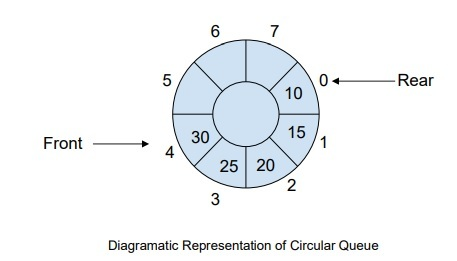# How to Manage Full Circular Queue Event in C++?

## Introduction

Circular Queue is an improvement over a linear queue and it was introduced to address the memory wastage problem in the linear queue. A circular queue uses the FIFO principle for the insertion and removal of the elements from it. In this tutorial, we will discuss the operations of the circular queue and how to manage it.

## What is Circular Queue?

A circular queue is another kind of queue in a data structure, whose front and rear ends are connected with each other. It is also known as Circular Buffer. Its operations are similar to the linear queue, so why do we need a new queue in the data structure?

With the linear queue, when the queue reaches its maximum limit, there is a possibility of some memory space before the rear point. It results in memory loss and a good algorithm is one that utilizes resources at their maximum.

To address the problem of memory wastage, developers introduced the concept of a circular queue with circular links to the rear end and front end and the possibility of inserting more elements.The basic functions of the Circular Queue

• Rear − It returns the rear value of the queue.

• Front − It returns the front value of the queue.

• deQueue − This in-built method is used to remove elements from the queue while checking if it is empty or not.

• enQueue − This method is used to insert new elements to the queue while checking its size.

In a circular queue, elements are added at the rear end and removed elements from the front end. deQueue and enQueue are queue-size-independent functions and use the modulo operator for their implementation. Their time complexity is O(1).

## Managing a Circular Queue

We are managing a circular queue by using the enQueue and deQueue operations. Initially, a circular queue has a front value of 0 and a rear value of -1, where all elements in the circular queue are NULL.

### Example

C++ code of circular Queue Implementation using an array

#include <bits/stdc++.h>
using namespace std;

class Queue {
//Initializing front and rear of the queue
int rear, front;
int sz;
int* arr;

public:
Queue(int s) {
front = rear = -1;
sz = s;
arr = new int[s];
}

void enQueue(int v);
int deQueue();
void displayQueue();
};

//Circular queue function
void Queue::enQueue(int v) {
if ((front == 0 && rear == sz - 1)
|| (rear == (front - 1) % (sz - 1))) {
printf("\nNo Space Queue is Full");
return;
}

//Inserting the front element
else if (front == -1) {
front = rear = 0;
arr[rear] = v;
}

else if (rear == sz - 1 && front != 0) {
rear = 0;
arr[rear] = v;
}

else {
rear++;
arr[rear] = v;
}
}

//Function for deleting queue elements
int Queue::deQueue() {
if (front == -1) {
printf("\nQueue needs data it is empty");
return INT_MIN;
}

int ele = arr[front];
arr[front] = -1;
if (front == rear) {
front = -1;
rear = -1;
}
else if (front == sz - 1)
front = 0;
else
front++;
return ele;
}

//Printing Circular queue elements
void Queue::displayQueue() {
if (front == -1) {
printf("\nQueue Empty");
return;
}
printf("\nCircular Queue elements are: \n");
if (rear >= front) {
for (int i = front; i <= rear; i++)
printf("%d ", arr[i]);
} else {
for (int i = front; i < sz; i++)
printf("%d ", arr[i]);

for (int i = 0; i <= rear; i++)
printf("%d ", arr[i]);
}
}

int main() {
Queue q(5);
//Pushing data in circular queue
q.enQueue(10);
q.enQueue(20);
q.enQueue(3);
q.enQueue(5);
//Printing circular queue elements
q.displayQueue();

//Deleting front elements of circular queue
printf("\nDeleted element = %d\n", q.deQueue());
printf("\nDeleted element = %d", q.deQueue());
q.displayQueue();
q.enQueue(13);
q.enQueue(27);
q.enQueue(50);
q.displayQueue();
q.enQueue(22);

return 0;
}


### Output

Circular Queue elements are:
10 20 3 5
Deleted element = 10

Deleted element = 20
Circular Queue elements are:
3 5
Circular Queue elements are:
3 5 13 27 50
No Space Queue is Full


## Conclusion

A circular queue is used in memory management and CPU scheduling. It uses the displayQueue() function to display the queue elements.

We reached the end of this tutorial. I hope this tutorial helped you to understand how to implement a circular Queue.

Updated on: 22-Feb-2023

107 Views